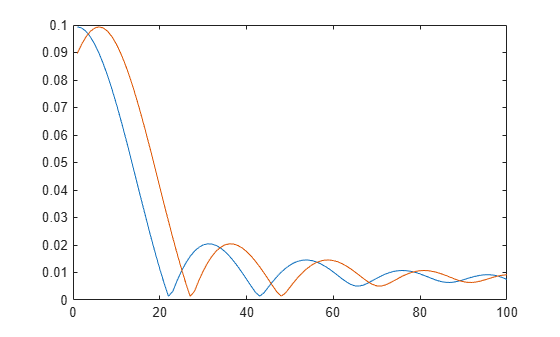Main Content

# lteSLFrameOffsetPSSCH

PSSCH DM-RS sidelink subframe timing estimate

## Syntax

``offset = lteSLFrameOffsetPSSCH(ue,waveform)``
``````[offset,corr] = lteSLFrameOffsetPSSCH(ue,waveform)``````

## Description

example

````offset = lteSLFrameOffsetPSSCH(ue,waveform)` performs synchronization using PSSCH demodulation reference signal (DM-RS) symbols for the time-domain waveform, `waveform`, given UE-specific settings, `ue`.The returned `offset` indicates the number of samples from the start of the input waveform to the position in that waveform where the first subframe containing DM-RS begins.```

example

``````[offset,corr] = lteSLFrameOffsetPSSCH(ue,waveform)``` also returns a complex matrix, `corr`, which is used to extract the timing offset.```

## Examples

collapse all

Synchronize and demodulate a transmission that has been delayed by five samples. The transmission contains PSSCH demodulation reference signal (DM-RS) symbols that are used when estimating the waveform timing offset.

Create a UE configuration specifying 15 resource blocks, a sidelink identity of 1, a normal cyclic prefix, a PSSCH subframe number of 0, and a `PRBSet` of 1.

```ue = struct('NSLRB',15,'NSAID',1,'CyclicPrefixSL','Normal', ... 'NSubframePSSCH',0,'PRBSet',1);```

Create a resource grid and modulate the waveform containing PSSCH DM-RS symbols.

```txgrid = lteSLResourceGrid(ue); txgrid(ltePSSCHDRSIndices(ue)) = ltePSSCHDRS(ue); txwaveform = lteSLSCFDMAModulate(ue,txgrid);```

Add a time delay of five samples.

`rxwaveform = [zeros(5,1); txwaveform];`

Calculate the timing offset in samples.

`offset = lteSLFrameOffsetPSSCH(ue,rxwaveform)`
```offset = 5 ```

Correct the timing offset and demodulate the received waveform.

`rxGrid = lteSLSCFDMADemodulate(ue,rxwaveform(1+offset:end));`

View the correlation peak for a transmission waveform that has been delayed by five samples. The transmission contains PSSCH demodulation reference signal (DM-RS) symbols available for estimating the waveform timing.

Create a UE configuration specifying 15 resource blocks, a sidelink identity of 1, a normal cyclic prefix, a PSSCH subframe number of 0, and a `PRBSet` of 1.

```ue = struct('NSLRB',15,'NSAID',1,'CyclicPrefixSL','Normal', ... 'NSubframePSSCH',0,'PRBSet',1);```

Create a resource grid and modulate the waveform containing PSSCH DM-RS symbols.

```txgrid = lteSLResourceGrid(ue); txgrid(ltePSSCHDRSIndices(ue)) = ltePSSCHDRS(ue); txwaveform = lteSLSCFDMAModulate(ue,txgrid);```

Calculate the timing offset in samples.

`[offset corr] = lteSLFrameOffsetPSSCH(ue,txwaveform);`

Add a time delay of five samples.

`rxwaveform = [zeros(5,1); txwaveform];`

Calculate the timing offset in samples.

`[offset corrDelayed] = lteSLFrameOffsetPSSCH(ue,rxwaveform);`

Plot the correlation data before and after delay is added. Zoom in on the x-axis to view correlation peaks.

```plot(corr) hold on plot(corrDelayed) hold off xlim([0 100])```Correct the timing offset and demodulate the received waveform.

`rxGrid = lteSLSCFDMADemodulate(ue,rxwaveform(1+offset:end));`

## Input Arguments

collapse all

User equipment settings, specified as a parameter structure containing these fields:

Sidelink mode, specified as `'D2D'` or `'V2X'`.

Data Types: `char` | `string`

Number of sidelink resource blocks, specified as an integer scalar from 6 to 110.

Example: `6`, which corresponds to a channel bandwidth of 1.4 MHz.

Data Types: `double`

Sidelink group destination identity, specified as an integer in the interval [0, 255].

This field is the lower eight bits of the full 24-bit ProSe Layer-2 group destination ID. This field and the `NSubframePSSCH` field control the value of the scrambling sequence at the start of each subframe. This field is required only for D2D sidelink.

Data Types: `double`

V2X scrambling identity, specified as an integer scalar. `NXID` is the 16 bit CRC associated with the PSCCH SCI grant. It is only required for V2X sidelink.

Data Types: `double`

Cyclic prefix length, specified as `'Normal'` or `'Extended'`.

Data Types: `char` | `string`

PSSCH subframe number in the PSSCH subframe pool, specified as an integer scalar. (${n}_{\text{ssf}}^{\text{PSSCH}}$)

`NSubframePSSCH` and `NSAID` control the values of the scrambling sequence. It is only required for D2D sidelink.

Data Types: `double`

Zero-based physical resource block (PRB) indices, specified as an integer column vector or a two-column integer matrix.

The PSSCH is intended to be transmitted in the same PRB in each slot of a subframe. Therefore, specifying `PRBSet` as a single column of PRB indices is recommended. However, for a nonstandard slot-hopping PRB allocation, `PRBSet` can be specified as a two-column matrix of indices corresponding to slot-wise resource allocations for PSSCH.

Data Types: `double`

Data Types: `struct`

Modulated sidelink waveform, specified as an NS-by-NR numeric matrix, where NS is the number of time-domain samples and NR is the number of receive antennas. `waveform` should be at least one subframe long and contain the DM-RS signals.

You can generate this matrix by performing SC-FDMA modulation on a resource matrix. To perform this modulation, use the `lteSLSCFDMAModulate` function or one of the channel model functions, such as `lteFadingChannel` or `lteMovingChannel`.

Data Types: `double`
Complex Number Support: Yes

## Output Arguments

collapse all

Offset number of samples, returned as a scalar integer. This output is the number of samples from the start of the waveform to the position in that waveform where the first subframe containing the DM-RS begins. `offset` is computed by extracting the timing of the peak of the correlation between `waveform` and internally generated reference waveforms containing DM-RS signals. The correlation is performed separately for each antenna. The antenna with the strongest correlation is used to compute `offset`.

Note

`offset` is the position of `mod(max(abs(corr),LSF))`, where LSF is the subframe length.

Signal used to extract the timing offset, returned as a complex numeric matrix. `corr` has the same dimensions as `waveform`.

## See Also

Introduced in R2017a